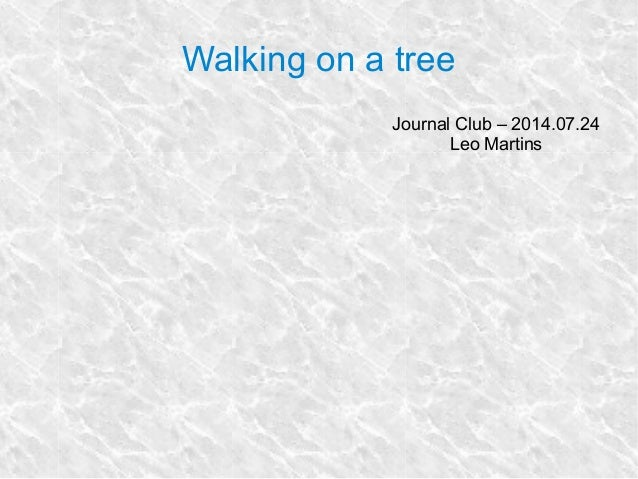Successfully reported this slideshow.
We use your LinkedIn profile and activity data to personalize ads and to show you more relevant ads. You can change your ad preferences anytime.Upcoming SlideShare
×

# Walking on a tree

425 views

Published on

Phylogenetic contrasts and other Markov Models on phylogenies
-- Presentation given at UVIGO in July/2014

Published in: Science
• Full Name
Comment goes here.

Are you sure you want to Yes No
Your message goes here• Be the first to comment

• Be the first to like this

### Walking on a tree

1. 1. Walking on a tree Journal Club – 2014.07.24 Leo Martins
2. 2. Problem: compare e.g. body mass (X) with speed (Y)
3. 3. Problem: compare e.g. body mass (X) with speed (Y)
4. 4. Problem: compare e.g. body mass (X) with speed (Y) Correlation between traits red and yellow is spurious Correlation between traits red and yellow is significant
5. 5. Solution: for each trait, calculate the constrasts
6. 6. Solution: for each trait, calculate the constrasts Brownian motion
7. 7. Solution: for each trait, calculate the constrasts Brownian motion
8. 8. Solution: for each trait, calculate the constrasts Brownian motion
9. 9. Solution: for each trait, calculate the constrasts Brownian motion
10. 10. Now we know what went wrong
11. 11. Other Markov processes on trees
12. 12. Other Markov processes on trees
13. 13. Other Markov processes on trees
14. 14. Other Markov processes on trees
15. 15. Other Markov processes on trees